# Torque

Torque and Mari each multiplied 1/8 inch times 5/8 inch. Tartaric 5/8 squares point inches. And Marie got 5/64 squared thought inches tall. Which student found a corrupt area?

x =  0.0781

### Step-by-step explanation: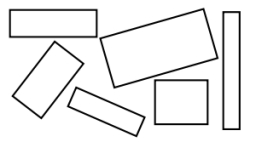Did you find an error or inaccuracy? Feel free to write us. Thank you!Tips to related online calculators
Need help to calculate sum, simplify or multiply fractions? Try our fraction calculator.

#### You need to know the following knowledge to solve this word math problem:

We encourage you to watch this tutorial video on this math problem:

## Related math problems and questions:

• Metal rodYou have a metal rod that’s 51/64 inches long. The rod needs to be trimmed. You cut 1/64 inches from one end and 1/32 inches from the other end. Next, you cut the rod into 6 equal pieces. What will be the final length of each piece?
• TimothyTimothy is 56 4/5 inches tall. Theresa is 1 1/6 inches shorter than Timothy and Jane is 1 1/3 inches shorter than Theresa. How tall is Jane?
• A rectangle 6A rectangle measures 2 4/5 inches by 2 1/5 inches. What is its area?
• Max vs SharonMax is 1 1/2 times as tall as Sharon. Alan is 4 1/3 feet tall. If Alan is 6 inches taller than Sharon, how tall is Max?
• Length 7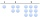Length equals 7/6 inch in width equals 7/9 inches the area is?
• Jewelry box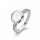The bottom of Zeyda's jewelry box is a rectangle with the length of 5 3/8 inches and a width of 3 1/4 inches. What is the area of the bottom of the jewelry box?
• Thomas 2Thomas is 56 3/4 inches tall. Jillian is 1 3/8 inches shorter than Thomas and Jane is 1 1/5 inches shorter than Jillian. How tall is Jane? Label the names on the tape diagram to represent this problem. Jane Jillian Thomas Excellent!
• Milo liftedMilo lifted 65 lb 6 times and Jeremy lifted 50 lb 9 times. Which one lifted the most weight?
• Divide 11Divide the product of 4 and 5/8 by 1 1/2. Write your answer as a mixed number.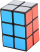A gift box has dimensions of: 8 1/2 inches, 5 1/2 inches, and 2 1/2 inches, respectively. How many cubes with side lengths of 1/2 inches would be needed to fill the gift box?
• CompareCompare fractions (34)/(3) and (12)/(4). Which fraction of the lower?
• Evaluate expressionEvaluate expression using BODMAS rule: 1 1/4+1 1/5÷3/5-5/8
• Fractions and mixed numerals(a) Convert the following mixed numbers to improper fractions. i. 3 5/8 ii. 7 7/6 (b) Convert the following improper fraction to mixed number. i. 13/4 ii. 78/5 (c) Simplify these fractions to their lowest terms. i. 36/42 ii. 27/45 2. evaluate following ex
• Tallest and shortestJenn is the tallest on the team. He is 1 1/2 times as tall as the shortest girl whom is 4 1/4 feet tall. How tall is Jebb?
• Fraction of a NumberIf 1/2 of 1/3 of 1/4 of 1/5 of a number is 2.5. what is the number?
• Find the 28Find the area of a square with side length 4/5 yard.
• Oceans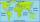The Earth's surface is approximately 510,000,000 km2 and is 7/10 covered by oceans. Of which 1/2 covers the Pacific Ocean, the Atlantic Ocean 1/4, the Indian Ocean 1/5 and the Arctic Ocean 1/20. What parts of the Earth's surface cover each ocean?